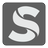Area of a Convex Polygon
Simple+
English ES RU

There is a convex polygon on a coordinate plane. This polygon is presented as a list of vertices coordinates. Each vertex is connected sequentially with the last connecting back to the first. A polygon has N vertices. You should write a program that will calculate the area of a polygon. The result should be given with one digits precision as ±0.1.Input: Coordinates as a list of lists. Each list contains two integers.

Output: The area of the polygon as a float.

Example:

```checkio([[1, 1], [9, 9], [9, 1]]) == 32
checkio([[4, 10], [7, 1],...```
You should be an authorized user in order to see the full description and start solving this mission.